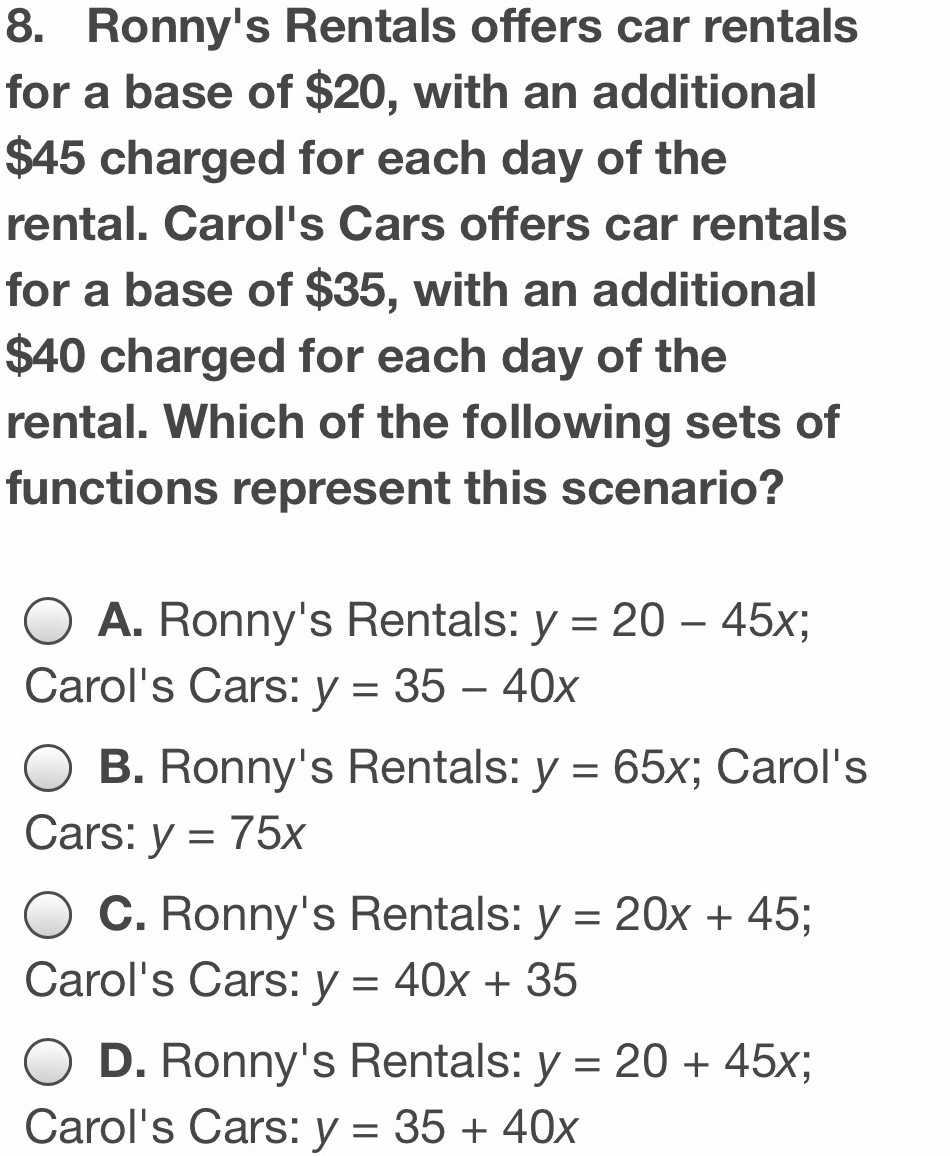### ¿Todavía tienes preguntas de matemáticas?

Pregunte a nuestros tutores expertos
Algebra
Pregunta8. Ronny's Rentals offers car rentals for a base of $$\ 20 ,$$ with an additional $$\ 45$$ charged for each day of the rental. Carol's Cars offers car rentals for a base of $$\ 35 ,$$ with an additional $$\ 40$$ charged for each day of the rental. Which of the following sets of functions represent this scenario?

A. Ronny's Rentals: $$y = 20 - 45 x ;$$

Carol's Cars: $$y = 35 - 40 x$$

B. Ronny's Rentals: $$y = 65 x ;$$ Carol's Cars: $$y = 75 x$$

C. Ronny's Rentals: $$y = 20 x + 45$$ ; Carol's Cars: $$y = 40 x + 35$$

D. Ronny's Rentals: $$y = 20 + 45 x$$ ; Carol's Cars: $$y = 35 + 40 x$$